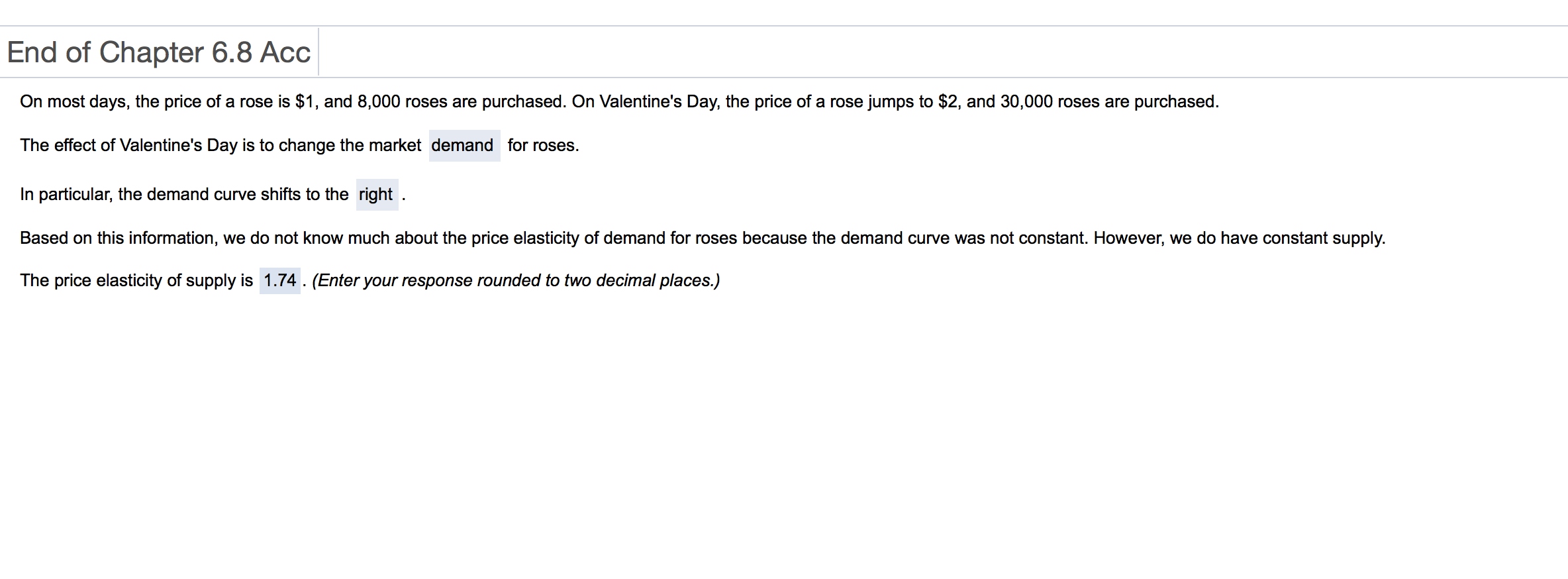# End of Chapter 6.8 Acc On most days, the price of a rose is $1, and 8,000 roses are purchased. On Valentine's Day, the price of a rose jumps to$2, and 30,000 roses are purchased The effect of Valentine's Day is to change the market demand for roses. In particular, the demand curve shifts to the right Based on this information, we do not know much about the price elasticity of demand for roses because the demand curve was not constant. However, we do have constant supply. The price elasticity of supply is 1.74. (Enter your response rounded to two decimal places.)

Question
1899 views

not sure hot to get to final answerhelp_outlineImage TranscriptioncloseEnd of Chapter 6.8 Acc On most days, the price of a rose is $1, and 8,000 roses are purchased. On Valentine's Day, the price of a rose jumps to$2, and 30,000 roses are purchased The effect of Valentine's Day is to change the market demand for roses. In particular, the demand curve shifts to the right Based on this information, we do not know much about the price elasticity of demand for roses because the demand curve was not constant. However, we do have constant supply. The price elasticity of supply is 1.74. (Enter your response rounded to two decimal places.) fullscreen

1 Rating

### Want to see the step-by-step answer?